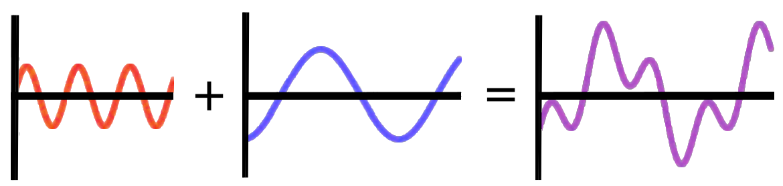# Periodic Plus Periodic

Algebra Level 2True or False:

Given any two periodic functions, $\color{#D61F06}{f(x)}$ and $\color{#3D99F6}{g(x)}$, the function $\color{#69047E}{h(x)} = \color{#D61F06}{f(x)} + \color{#3D99F6}{g(x)}$ will also be periodic with a period that is, at most, the product of the original two periods (the period of $f(x)$ times the period of $g(x)$).

×

Problem Loading...

Note Loading...

Set Loading...